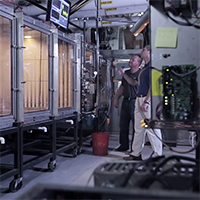# Calculating Natural and Mechanical Loads: Humidification Basics Part 4

Norm Hall, R.L. Deppmann and Chad Edmondson, J. M. Pleasants
/
September 26, 2016

How do we determine how much moisture should be added to the air for a given application? In other words, how do we calculate the humidification load for a given building?

The basis of this calculation is the same, whether you intend on using natural, mechanical, or economizer cycle ventilation. Natural and mechanical ventilation methods are virtually the same, which is why we’ve chosen to cover both in this blog. Economizer cycle is a bit more complex, so we’ll cover that as a stand-alone topic in our next blog.

Natural ventilation relies on natural (not mechanical) forces to regulate the flow of air through a building via windows, vents, or other openings. There is no fan involved. An example might be a livestock facility, or, at a more sophisticated level, a high-performance green building. Mechanical ventilation, however, relies on a fan to supply fresh air to the space.## Calculating Humidification for Natural Ventilation

Regardless of the ventilation method, calculating humidification load always begins with knowing (or estimating) how much air will be introduced into the space. Most non-critical, naturally-ventilated designs are based on 1 to 2 air changes per hour (ACH). If the space is very open, you may use 2 ACH; if it is fairly tight, you may use 1 ACH.

So let’s say we have a very tight structure with 115,200 cubic feet of space and an ACH of 1. The desired conditions are 70°F at 50% relative humidity (RH). The entering air design conditions are 10°F and 45% RH.

By consulting a psychrometric chart or table, we know that air at 10°F and 45% RH has a moisture content of 0.30 lb per hour per 100 cfm. The same psychrometric chart tells us that the moisture content of the air we hope to achieve (70°F at 50% RH) is 3.44 lb per hour per 100 cfm. The difference between the two is 3.14 lb, which is the amount of moisture we must add to the air to achieve our desired indoor conditions.

Next we use the following formula to calculate the CFM:

CFM = No. of air changes/hr x cubic volume ÷ 60 minutes

Or, in the case of our example:

(1 ACH x 115,200 ft.) ÷ 60 min per hour = 1,920 cfm.

Finally, we determine the humidification load by multiplying the CFM load by the amount of moisture that needs to be added to the air:

[1,920 cfm ÷ 100 cfm] × 3.14 lb per hour = 60.29 lb per hour

(Note: Remember the moisture content is based on lbs. per hour per 100 CFM, which is why we divide by CFM)

Thus, our humidification load is 60.29 lb per hour.

## Calculating Humidification for Mechanical Ventilation

To determine the humidification load for a mechanically vented space, you follow the same steps as above, but rather than using a factor of 1 to 2 ACH, we have to incorporate the percentage of outside air to total circulated air. To do this, we multiply the total air circulated by the percentage of outside air being introduced into the building. So if we have a mechanical ventilation system that circulates air at 9000 cfm with 25% outside air, our calculation would be:

9,000 cfm × 25 percent = 2,250 cfm.

Using this CFM, we then calculate humidification load as we did before:

[2,250 cfm ÷ 100 cfm] × 3.14 lb per hour = 70.65 lb per hour.

For most applications, as a general rule of thumb, expect around 3 pounds per hour for every 100 CFM of outside air that is introduced. Next up, will apply these principles to airside economizer applications!